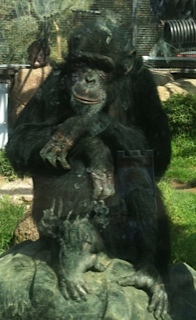# BrainPhooD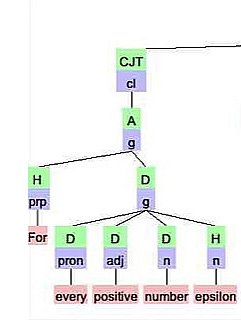# Parsing Mathematical Text

During the last few years I have been working on a project involving Natural Language Processing (NLP). On Thursday, March 8, 2012 I presented my ideas on this to an AI class at CSUEB. Here is an abstract and here are the slides.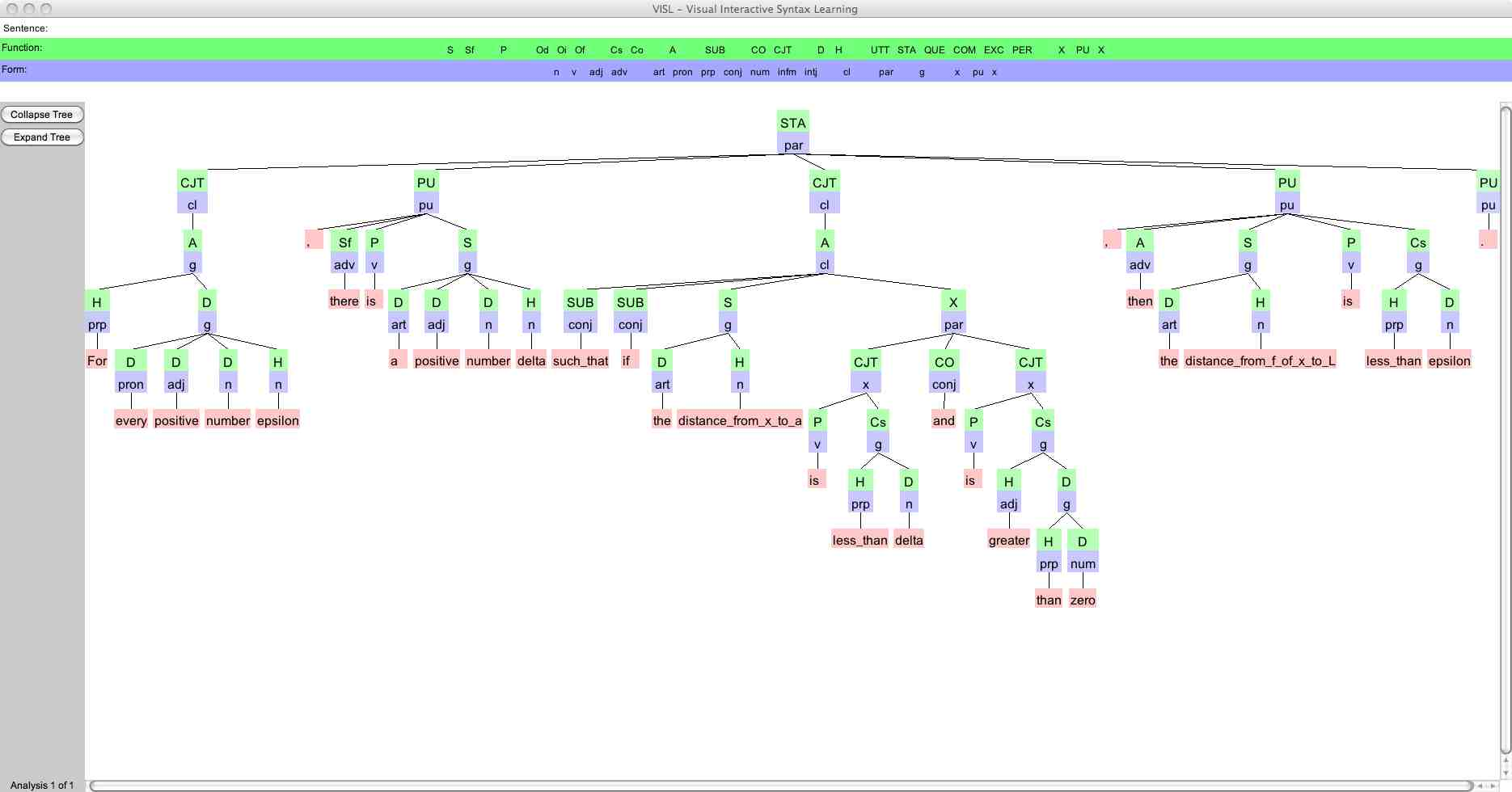This is the definition of limit of a function at a point, which is denoted by: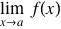It was obtained by submitting the text "For every positive number epsilon..." to the VISL parser at the South Danish University Arboretum web site.
This site handles a variety of different languages, including English.

The expression can be parsed by compilers or interpreters.
For example, here is what Mathematica does with it: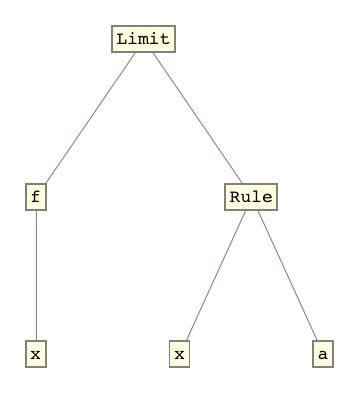The  mathematical text diagramed above appears in some form in most modern Calculus textbooks.
It is often called the precise definition of limit of a function at a point.
The basic idea is arguably attributed to Agustin-Louis Cauchy.
However, it is perhaps better to associate it with Karl Theodor Wilhelm Weierstrass, who was one of my academic ancestors according to a chart generated by The Mathematics Genealogy Project.

This output clearly illustrates the separation of function and form for each grammatical group.
Each grammatical group is represented by a node in the graph.
Each node is divided into an upper part containing the label for the function of the node (and its corresponding grammatical group) and a lower part containing a label for the form of the branch headed by that node (and its corresponding grammatical group).
This diagram also illustrates how to cause the parser to treat a group as one word.
We do this by jamming the words together, replacing all spaces by underscores.
A separate page can handle each such jammed group, displaying its grammatical structure for the unjammed form.
For example, the phrase "distance from x to a" is discussed on a page for distance.

I am not in complete agreement with the graph.
Here is the result of applying the Stanford parser.

One of my Calculus students consulted two members of the faculty in the Department of English at our university.
Here is the result of that effort.

One of my goals is to guide the development of a software system for processing mathematical text.
One of my computer science students, Arvind Punj, has completed a computer science masters thesis A Knowledge Management System for Mathematical Text under my direction that provides groundwork for such a system.

Some of my other masters thesis students are doing interesting stuff. For example, Ranbir Parmar has done his MS thesis along the lines of the following paper:

http://conferences.sigcomm.org/sigcomm/2015/pdf/papers/p57.pdf Hirochika Asai, The University of Tokyo, panda@hongo.wide.jp

The first sentence of this paper can be parsed as:

```&lt;s&gt; SOURCE: Running text
1. Internet of Things leads to routing table explosion.
A1
STA:cl(fcl) .
|-S:n('Internet_of_Things' &lt;heur&gt; S NOM)	Internet_of_Things
|-Op:g(pp)
|-H:prp('to')	to
|-D:g(np)